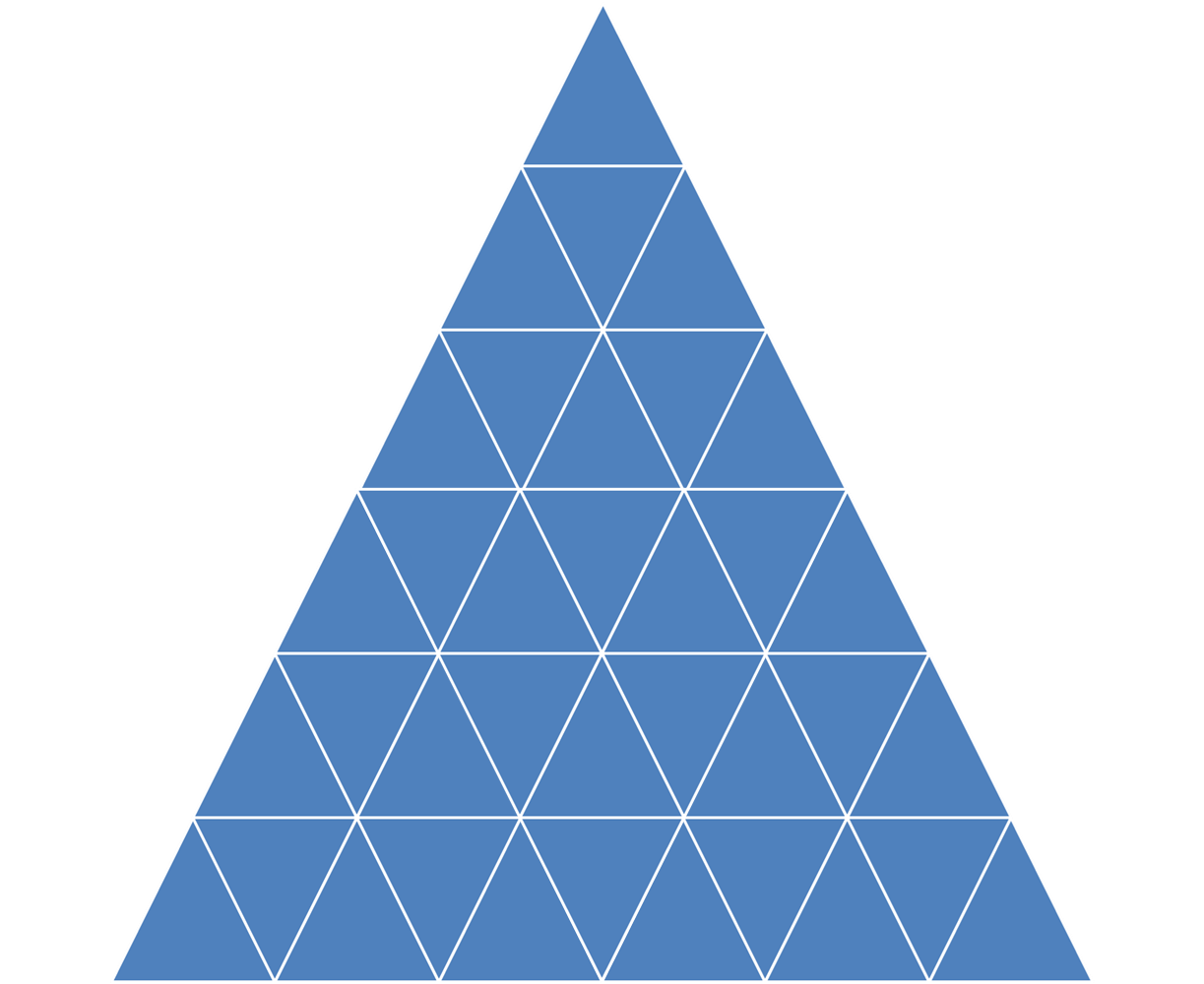# How many Triangles?This pattern has 6 levels of triangles. What is the total number of triangles in this pattern? Similarly, try to find out a general solution for total number of triangles where number of levels is even more.

×